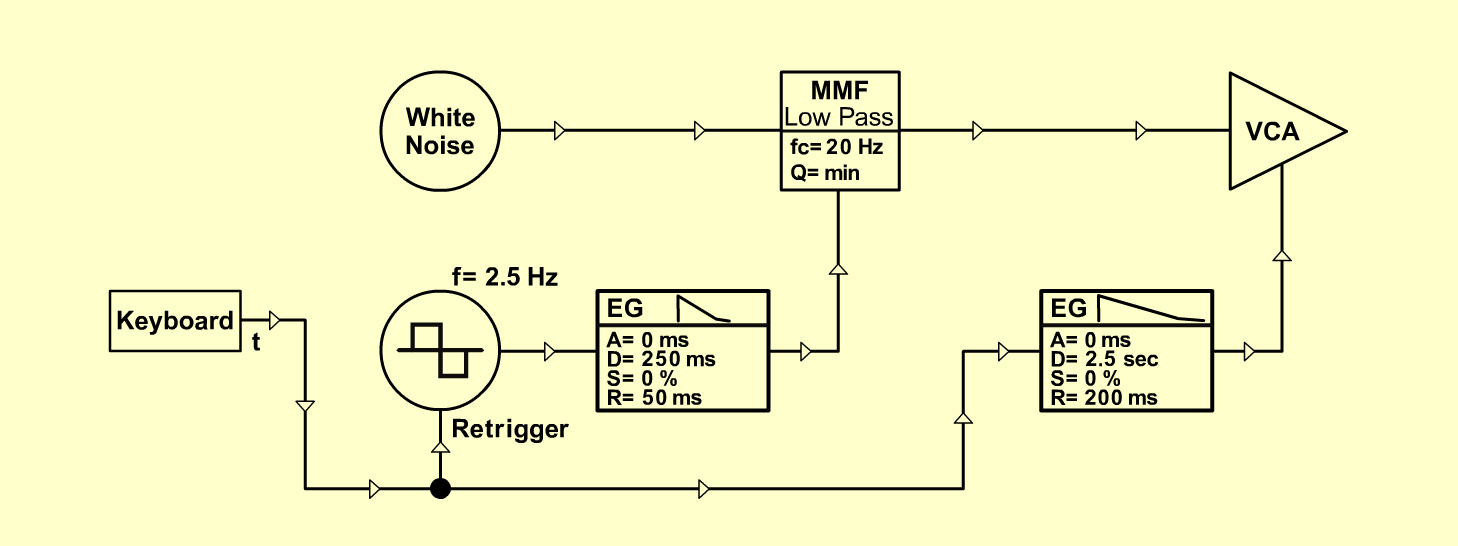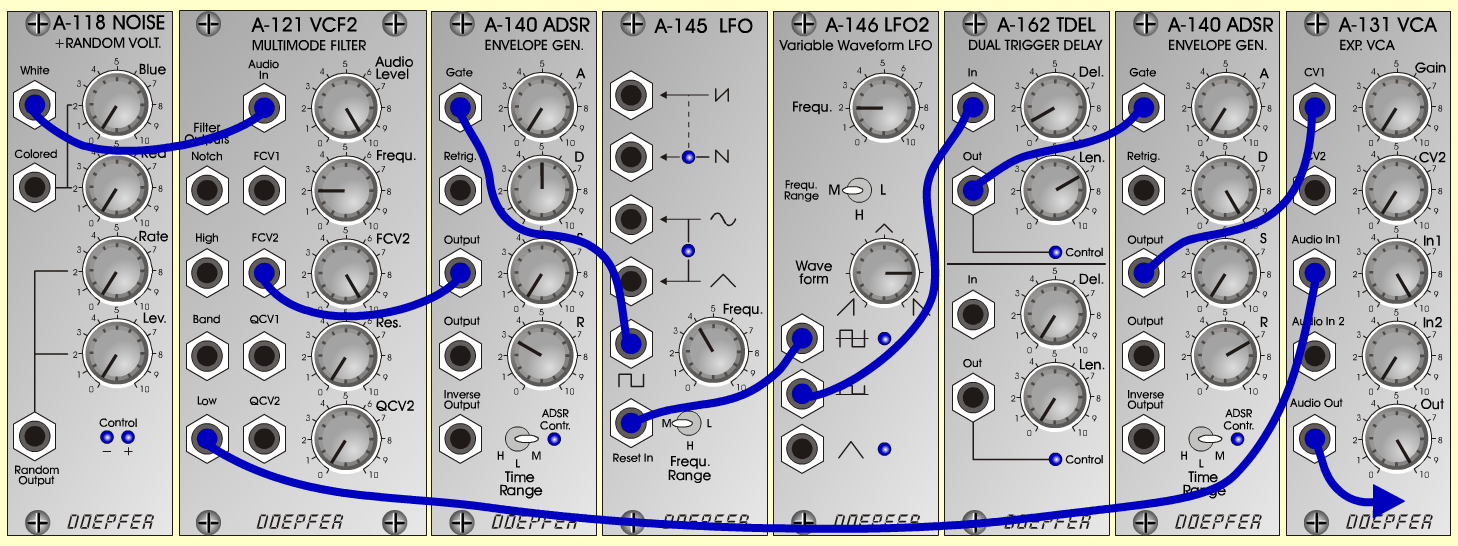I's ForgeFXD o e p f e r A - 100 Connections: Settings: A-118 (White) <=> A-121 (Audio In) A-121 (Low) <=> A-131 (Audio In) A-145 (Square) <=> A-140/1 (Gate) A-146 (Square pos) <=> A-162 (In) A-146 (Square) <=> A-145 (Reset In) A-162 (Out) <=> A-140/2 (Gate) A-131 (Audio Out)  <=> Amplifier A-121 (Audio Level = 10, Freq = 2, FCV 2 = 10, Res = 0) A-131 (Gain = 0, Audio In 1 = 10, Audio Out = 10) A-140/1 (A = 0, D = 5, S = 0, R = 3, Range = M) A-140/2 (A = 0, D = 10, S = 0, R = 7, Range = M) A-145 (Frq = 4, Range = M) A-146 (Freq = 2, Wave Form = 8, Range = M) A-162 (Del = 1, Len = 7) Notes: With Colored noise: A-118 (Blue = 0, Red = 10) Change the fc: A-121 (Freq = 3 / Freq = 4)>I's forge< stands for >Isengart's forge<. Josef MuellerSound samples I's forge 1 I's forge 2 I's forge 3 I's forge 4 I's forge 5 I's forge 6 I's forge 7 I's forge 8